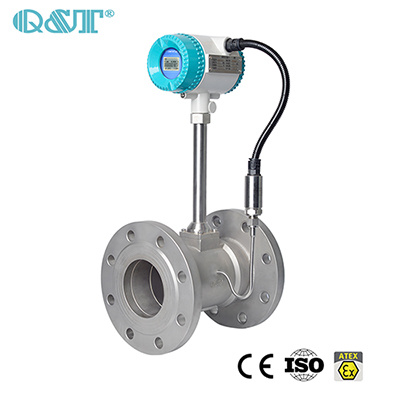vortex flow meter conversion method of working condition & standard condition

2023-02-02
Vortex flow meter conversion method of working condition & standard condition

In the production process of the vortex flow meter, we need to determine an important parameter: the flow parameter; the flow range of the vortex flow meter is basically fixed, and the selection is based on the flow rate of the working condition. Knowing the standard condition of our street flow meter, how do we calculate the working condition flow rate to choose the caliber.
The installation conditions of the vortex flow meter below DN300 are: pipeline type, and above DN300 are: plug-in type, which can be used to detect the flow of various liquid and gas media, not only the volume flow of the medium but also the mass flow of the medium. It is very important to choose the installation point of the vortex flow meter correctly and install the flow meter correctly. If the installation link is wrong, it will affect the measurement accuracy and even damage the vortex flow meter.
The Instrument coefficient of vortex flow meter: It has been debugged and tested before leaving the factory. The instrument constant K of each flow meter has been indicated on the nameplate and the factory certificate. Its physical meaning is in the calibrated state (P=101.3kPa , t=20°C) per 1 liter of volume flow through the flow meter, the number of pulses output by the flow meter, the unit is 1/L. Due to the temperature change of the measurement medium, the geometric size of the measurement pipe and the vortex generator changes (thermal expansion and contraction), the flow instrument constant of the vortex flow meter needs to be corrected, and the expression of the correction coefficient KT is: KT=1-4.8× 10-5×(t-20) where: t──The temperature of the measuring medium, °C.
Vortex flow meter working condition volume flow conversion method:

(1): According to the flow range of the vortex flow meter set by the process, the volume flow rate under the actual pipeline working state is calculated:

The flow rate set by the process can be mass flow rate (kg/h), volume flow rate under working condition (m3/h) or volume flow rate under standard condition (N m3/h), and the mass flow rate or volume flow rate under standard condition can be converted into The method of

volume flow in working condition is as follows:

a. The vortex flowmeter converts the maximum flow rate (range upper limit) Gmax (kg/h) of the mass flow rate into the volume flow rate Qmax (m3/h) in the working state, and the calculation formula is:

Qmax= Gmax×─ (m3/h)

In the formula: ρ──The density of the medium under the working conditions of the instrument (kg/m3)

b. The maximum flow rate (range upper limit) Q0max (Nm3/h) of the gas standard state is converted into the volume flow rate Qmax (m3/h) under the working state, and the calculation formula is:

Qmax= Q0max×──────×────── (m3/h

In the formula: P──Gauge pressure of the gas under the working conditions of the instrument (MPa);

t──The temperature of the gas under the working conditions of the instrument (°C).

(2): Calculate the maximum frequency fmax of the vortex street according to the maximum volume flow Qmax (m3/h) in the working state:

fmax = ───×Qmax×K×KT (Hz)

In the formula: K──instrument coefficient (1/L), the K value is marked on the instrument nameplate;

KT——Temperature correction coefficient.

If a valve is installed near the upstream of the installation point of the vortex flowmeter, and the valve is continuously opened and closed, it will have a great impact on the service life of the flowmeter, and it is very easy to cause permanent damage to the flowmeter. Try to avoid installing the flowmeter on a very long overhead pipeline. After a long time, the sagging of the vortex flowmeter will easily cause the seal leakage of the flowmeter on the flange. Pipeline fastening devices are set at the upstream and downstream 2D positions respectively.share: